U.S. Department of Transportation
1200 New Jersey Avenue, SE
Washington, DC 20590
202-366-4000

Federal Highway Administration Research and Technology
Coordinating, Developing, and Delivering Highway Transportation InnovationsThis report is an archived publication and may contain dated technical, contact, and link information
 Federal Highway Administration > Publications > Research Publications > 04097 > Measured Variability Of Southern Yellow Pine - Manual for LS-DYNA Wood Material Model 143
Publication Number: FHWA-HRT-04-097
Date: August 2007

# Measured Variability Of Southern Yellow Pine - Manual for LS-DYNA Wood Material Model 143

PDF files can be viewed with the Acrobat® Reader®

### 1.5 PLASTIC FLOW

The plasticity algorithms limit the stress components once the failure criterion in equations 13 or 14 is satisfied. This is done by returning the stress state back to the yield surface.1

Our traditional approach for modeling plasticity is to partition the stress and strain tensors into elastic and plastic parts. Partitioning is done with return mapping algorithms that enforce the plastic consistency conditions. Such algorithms allow one to control plastic strain generation. In addition, return mapping algorithms with associated flow satisfy the second law of thermodynamics. Associated flow is discussed in appendix D.

1Two simple types of plasticity algorithms are those that reduce the moduli directly and those that scale back the stresses directly. Although simple to implement, such methods do not allow one to control plastic strain generation and do not necessarily satisfy the second law of thermodynamics.

Separate plasticity algorithms are modeled for the parallel and perpendicular modes by enforcing separate consistency conditions. Each consistency condition is derived in appendix D. The solution of each consistency condition determines the consistency parameters Dlúú and Dl^. The Dl solutions, in turn, determine the stress updates.

Parallel Modes

The parallel failure criterion from equation 13 is redefined as f|| (I1, I4) ≥ 0, with:

where I1 and I4 are two of the five invariants of a transversely isotropic material. In this case, the expression Dl|| is:

Dl|| is calculated from specification of the total strain increments and the yield function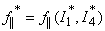. The trial elastic stress invariants,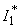and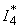, are calculated from the trial elastic stresses (see section 1.5.2). For the failure surface function in equation 15, the partial derivatives in equation 16 are: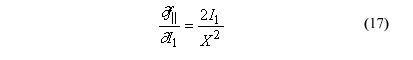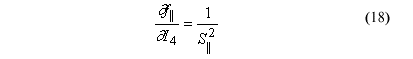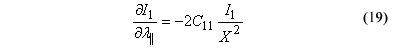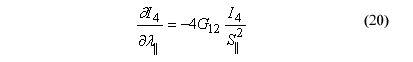Perpendicular Modes

The perpendicular failure criterion from equation 14 is redefined as f^ (I3, I4) ≥ 0, with:

where I2 and I3 are two of the five invariants of a transversely isotropic material. In this case, the expression for Dl^ is:

Dl^is calculated from specification of the total strain increments and the yield function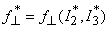, where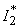and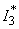are the trial elastic stress invariants. For the failure surface function in equation 21, the partial derivatives in equation 22 are:

The stresses are readily updated from the total strain increments and the consistency parameters: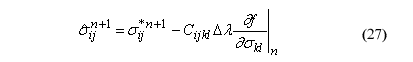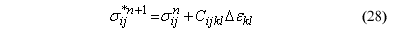where:
n       = nth time step in the finite element analysis
s*ij   = trial elastic stress updates calculated from the total strain increments, Deij, prior to application of plasticity

Total strain increments are calculated by the finite element code from the dynamic equations of motion and the time step.

Each normal stress update depends on the consistency parameters and failure surface functions for both the parallel (Dl = Dl|| and f = f||) and perpendicular (Dl = Dl^ and f = f^) modes. Each shear stress update depends on just one consistency parameter and failure surface function. If neither parallel nor perpendicular yielding occurs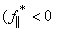and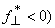, then Dl = 0 and the stress update is trivial: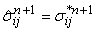.

For the stress updates in equation 27, the partial derivatives are:

Federal Highway Administration | 1200 New Jersey Avenue, SE | Washington, DC 20590 | 202-366-4000
Turner-Fairbank Highway Research Center | 6300 Georgetown Pike | McLean, VA | 22101